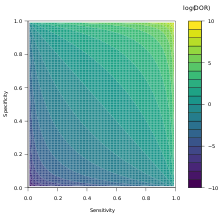# Diagnostic odds ratiolog(Diagnostic Odds Ratio) for varying sensitivity and specificity

In medical testing with binary classification, the diagnostic odds ratio is a measure of the effectiveness of a diagnostic test. It is defined as the ratio of the odds of the test being positive if the subject has a disease relative to the odds of the test being positive if the subject does not have the disease.

The rationale for the diagnostic odds ratio is that it is a single indicator of test performance (like accuracy and Youden's J statistic) but which is independent of prevalence (unlike accuracy) and is presented as an odds ratio, which is familiar to medical practitioners.

## Definition

The diagnostic odds ratio is defined mathematically as:where,,andare the number of true positives, false negatives, false positives and true negatives respectively.

### Confidence interval

As an odds ratio, the logarithm of the diagnostic odds ratio is approximately normally distributed. The standard error of the log diagnostic odds ratio is approximately:From this an approximate 95% confidence interval can be calculated for the log diagnostic odds ratio:Exponentiation of the approximate confidence interval for the log diagnostic odds ratio gives the approximate confidence interval for the diagnostic odds ratio.

## Interpretation

The diagnostic odds ratio ranges from zero to infinity, although for useful tests it is greater than one, and higher diagnostic odds ratios are indicative of better test performance. Diagnostic odds ratios less than one indicate that the test can be improved by simply inverting the outcome of the test – the test is in the wrong direction, while a diagnostic odds ratio of exactly one means that the test is equally likely to predict a positive outcome whatever the true condition – the test gives no information.

## Relation to other measures of diagnostic test accuracy

The diagnostic odds ratio may be expressed in terms of the sensitivity and specificity of the test:It may also be expressed in terms of the Positive predictive value (PPV) and Negative predictive value (NPV):It is also related to the likelihood ratios,and:## Uses

The log diagnostic odds ratio is sometimes used in meta-analyses of diagnostic test accuracy studies due to its simplicity (being approximately normally distributed).

Traditional meta-analytic techniques such as inverse-variance weighting can be used to combine log diagnostic odds ratios computed from a number of data sources to produce an overall diagnostic odds ratio for the test in question.

The log diagnostic odds ratio can also be used to study the trade-off between sensitivity and specificity. By expressing the log diagnostic odds ratio in terms of the logit of the true positive rate (sensitivity) and false positive rate (1 specificity), and by additionally constructing a measure,:It is then possible to fit a straight line,. If b ≠ 0 then there is a trend in diagnostic performance with threshold beyond the simple trade-off of sensitivity and specificity. The value a can be used to plot a summary ROC (SROC) curve.

## Example

Consider a test with the following 2×2 confusion matrix:

Condition (as determined by “Gold standard”)
Positive Negative
Test
outcome
Positive 26 12
Negative 3 48

We calculate the diagnostic odds ratio as:This diagnostic odds ratio is greater than one, so we know that the test is discriminating correctly. We compute the confidence interval for the diagnostic odds ratio of this test as [9, 134].

## Criticisms

The diagnostic odds ratio is undefined when the number of false negatives or false positives is zero – if both false negatives and false positives are zero, then the test is perfect, but if only one is, this ratio does not give a usable measure. The typical response to such a scenario is to add 0.5 to all cells in the contingency table, although this should not be seen as a correction as it introduces a bias to results. It is suggested that the adjustment is made to all contingency tables, even if there are no cells with zero entries.

2. (PDF) http://srdta.cochrane.org/sites/srdta.cochrane.org/files/uploads/Chapter%2010%20-%20Version%201.0.pdf. Missing or empty |title= (help)
7. Leeflang, M. M.; Deeks, J. J.; Gatsonis, C; Bossuyt, P. M.; Cochrane Diagnostic Test Accuracy Working Group (2008). "Systematic reviews of diagnostic test accuracy". Annals of Internal Medicine. 149 (12): 889–97. doi:10.7326/0003-4819-149-12-200812160-00008. PMC 2956514. PMID 19075208.